# 第十四节，TensorFlow中的反卷积，反池化操作以及gradients的使用

### 一 反卷积原理

def conv2d_transpose(value,
filter,
output_shape,
strides,
padding="SAME",
data_format="NHWC",
name=None):

• value:代表通过卷积操作之后的张量，一般用NHWC类型。如果是NHWC类型，形状[batch, height, width, in_channels]，如果是NCHW类型，形状为[batch, in_channels, height, width]。
• filter：代表卷积核，形状为[height, width, output_channels, in_channels]。
• output_shape：反卷积输出的张量形状，它必须是能够生成value参数的原数据的形状，如果输出形状不对，函数会报错。
• strides:代表原数据生成value时使用的步长。
• padding:代表原数据生成value时使用的填充方式，是用来检查输入形状和输出形状是否合规的。
• data_format: 'NHWC' and 'NCHW' 类型。
• name：名称。

### 二 反卷积实例

我们通过对模拟数据进行卷积核反卷积的操作，来比较卷积与反卷积中padding在SAME和VALID下的变化。先定义一个[1,4,4,1]的矩阵，矩阵里的元素值都为1，与滤波器大小为2x2，步长为2x2，分别使用padding为SAME和VALID两种情况生成卷积数据，然后将结果再进行反卷积运算，打印输出的结果

'''

'''
import tensorflow as tf
import numpy as np

#模拟数据
img = tf.Variable(tf.constant(1.0,shape=[1,4,4,1]))

kernel =tf.Variable(tf.constant([1.0,0,-1,-2],shape=[2,2,1,1]))

#分别进行VALID和SAME操作
conv =  tf.nn.conv2d(img,kernel,strides=[1,2,2,1],padding='VALID')
cons =  tf.nn.conv2d(img,kernel,strides=[1,2,2,1],padding='SAME')

#VALID填充计算方式 (n - f + 1)/s向上取整
print(conv.shape)
#SAME填充计算方式 n/s向上取整
print(cons.shape)

#在进行反卷积操作
contv = tf.nn.conv2d_transpose(conv,kernel,[1,4,4,1],strides=[1,2,2,1],padding='VALID')
conts = tf.nn.conv2d_transpose(cons,kernel,[1,4,4,1],strides=[1,2,2,1],padding='SAME')

with tf.Session() as sess:
sess.run(tf.global_variables_initializer())

print('kernel:\n',sess.run(kernel))
print('conv:\n',sess.run(conv))
print('cons:\n',sess.run(cons))
print('contv:\n',sess.run(contv))
print('conts:\n',sess.run(conts))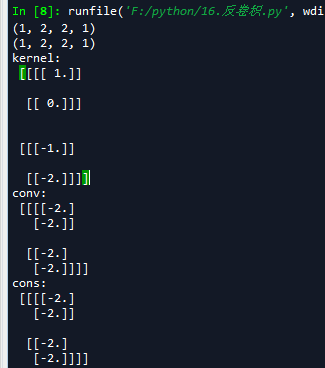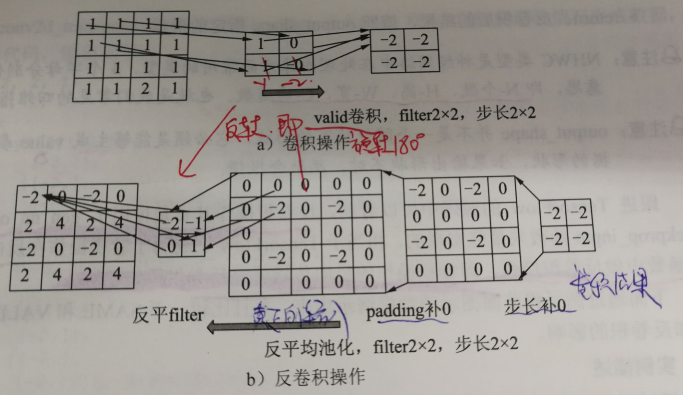### 三 反池化原理

• 平均池化比较简单。首先还原成原来的大小，然后将池化结果中的每个值都填入其对应于原始数据区域中的相应位置即可。如下图：
•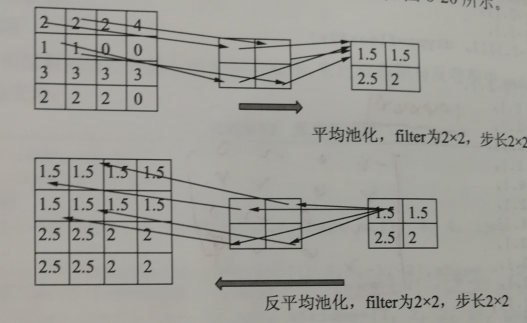• 最大池化的反池化会复杂一些。要求在池化过程中记录最大激活池的坐标位置，然后在反池化时，只把池化过程中最大激活值所在位置坐标的值激活，其它位置为0.当然，这个过程只是一种近似，因为在池化的过程中，除了最大值所在的位置，其他的值也是不为0的。如下图：
•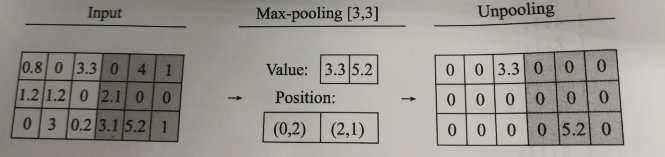### 四 反池化实例

TensorFlow中目前还没有反池化操作的函数。对于最大池化层，也不支持输出最大激活值得位置，但是同样有个池化的反向传播函数tf.nn.max_pool_with_argmax()。该函数可以找出位置，需要开发者利用这个函数做一些改动，自己封装一个最大池化操作，然后再根据mask写出反池化函数。

'''

'''

def max_pool_with_argmax(net,stride):
'''
重定义一个最大池化函数，返回最大池化结果以及每个最大值的位置(是个索引，形状和池化结果一致)

args:
net:输入数据 形状为[batch,in_height,in_width,in_channels]
stride：步长，是一个int32类型，注意在最大池化操作中我们设置窗口大小和步长大小是一样的
'''
#使用mask保存每个最大值的位置 这个函数只支持GPU操作
_, mask = tf.nn.max_pool_with_argmax( net,ksize=[1, stride, stride, 1], strides=[1, stride, stride, 1],padding='SAME')
#将反向传播的mask梯度计算停止
mask = tf.stop_gradient(mask)
#计算最大池化操作
net = tf.nn.max_pool(net, ksize=[1, stride, stride, 1],strides=[1, stride, stride, 1], padding='SAME')
#将池化结果和mask返回
return net,mask

def un_max_pool(net,mask,stride):
'''
定义一个反最大池化的函数，找到mask最大的索引，将max的值填到指定位置
args:
net:最大池化后的输出，形状为[batch, height, width, in_channels]
mask：位置索引组数组，形状和net一样
stride:步长，是一个int32类型，这里就是max_pool_with_argmax传入的stride参数
'''
ksize = [1, stride, stride, 1]
input_shape = net.get_shape().as_list()
#  calculation new shape
output_shape = (input_shape, input_shape * ksize, input_shape * ksize, input_shape)
# calculation indices for batch, height, width and feature maps
one_like_mask = tf.ones_like(mask)
batch_range = tf.reshape(tf.range(output_shape, dtype=tf.int64), shape=[input_shape, 1, 1, 1])
b = one_like_mask * batch_range
y = mask // (output_shape * output_shape)
x = mask % (output_shape * output_shape) // output_shape
feature_range = tf.range(output_shape, dtype=tf.int64)
f = one_like_mask * feature_range
# transpose indices & reshape update values to one dimension
updates_size = tf.size(net)
indices = tf.transpose(tf.reshape(tf.stack([b, y, x, f]), [4, updates_size]))
values = tf.reshape(net, [updates_size])
ret = tf.scatter_nd(indices, values, output_shape)
return ret

#定义一个形状为4x4x2的张量
img = tf.constant([
[[0.0,4.0],[0.0,4.0],[0.0,4.0],[0.0,4.0]],
[[1.0,5.0],[1.0,5.0],[1.0,5.0],[1.0,5.0]],
[[2.0,6.0],[2.0,6.0],[2.0,6.0],[2.0,6.0]],
[[3.0,7.0],[3.0,7.0],[3.0,7.0],[3.0,7.0]],
])

img = tf.reshape(img,[1,4,4,2])
#最大池化操作
pooling1 = tf.nn.max_pool(img,ksize=[1,2,2,1],strides=[1,2,2,1],padding='SAME')
#带有最大值位置的最大池化操作
pooling2,mask = max_pool_with_argmax(img,2)
#反最大池化
img2 = un_max_pool(pooling2,mask,2)
with tf.Session() as sess:
print('image:')
image = sess.run(img)
print(image)

#默认的最大池化输出
result = sess.run(pooling1)
print('max_pool:\n',result)

#带有最大值位置的最大池化输出
result,mask2 = sess.run([pooling2,mask])
print('max_pool_with_argmax:\n',result,mask2)

#反最大池化输出
result = sess.run(img2)
print('un_max_pool',result)

### 五 偏导计算

'''

'''
w1 = tf.Variable([[1,2]])       #1x2
w2 = tf.Variable([[3,4]])       #1x2

y = tf.matmul(w1,[,])    #2x1
#求w1的梯度
grads = tf.gradients(y,w1)      #1x2

with tf.Session() as sess:
sess.run(tf.global_variables_initializer())
gradval = sess.run(grads)
print(gradval)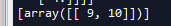import numpy as np
x = np.array([[9,10]])
print(x.shape)

x = np.array([,])
print(x.shape)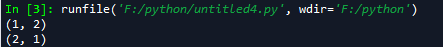tf.gradients()函数还可以同时对多个子式求关于多个变量的偏导：

tf.reset_default_graph()
w1 = tf.get_variable('w1',shape=)
w2 = tf.get_variable('w2',shape=)

w3 = tf.get_variable('w3',shape=)
w4 = tf.get_variable('w4',shape=)

y1 = w1 + w2 + w3
y2 = w3 + w4

gradients = tf.gradients([y1,y2],[w1,w2,w3,w4],grad_ys=[tf.convert_to_tensor([1.,2.]),tf.convert_to_tensor([3.,4.])])
with tf.Session() as sess:
sess.run(tf.global_variables_initializer())
gradval = sess.run(gradients)
print(gradval)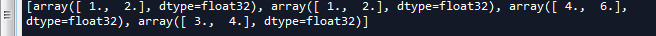### 六 梯度停止

'''

'''
w1 = tf.Variable(2.0)
w2 = tf.Variable(2.0)

a = tf.multiply(w1, 3.0)
#停止a节点梯度运算的功能
a_stoped = tf.stop_gradient(a)

b = tf.multiply(a_stoped, w2)
gradients = tf.gradients(b, xs=[w1, w2])
print(gradients)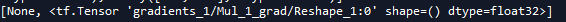a = tf.Variable(1.0)
b = tf.Variable(1.0)

c = tf.add(a, b)
#停止c节点梯度运算的功能
c_stoped = tf.stop_gradient(c)
d = tf.add(a, b)
e = tf.add(c_stoped, d)

gradients = tf.gradients(e, xs=[a, b])

with tf.Session() as sess:
tf.global_variables_initializer().run()
print(sess.run(gradients))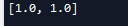# -*- coding: utf-8 -*-
"""
Created on Fri May  4 17:04:22 2018

@author: zy
"""

'''

'''
import tensorflow as tf
import numpy as np

#模拟数据
img = tf.Variable(tf.constant(1.0,shape=[1,4,4,1]))

kernel =tf.Variable(tf.constant([1.0,0,-1,-2],shape=[2,2,1,1]))

#分别进行VALID和SAME操作
conv =  tf.nn.conv2d(img,kernel,strides=[1,2,2,1],padding='VALID')
cons =  tf.nn.conv2d(img,kernel,strides=[1,2,2,1],padding='SAME')

#VALID填充计算方式 (n - f + 1)/s向上取整
print(conv.shape)
#SAME填充计算方式 n/s向上取整
print(cons.shape)

#在进行反卷积操作
contv = tf.nn.conv2d_transpose(conv,kernel,[1,4,4,1],strides=[1,2,2,1],padding='VALID')
conts = tf.nn.conv2d_transpose(cons,kernel,[1,4,4,1],strides=[1,2,2,1],padding='SAME')

with tf.Session() as sess:
sess.run(tf.global_variables_initializer())

print('kernel:\n',sess.run(kernel))
print('conv:\n',sess.run(conv))
print('cons:\n',sess.run(cons))
print('contv:\n',sess.run(contv))
print('conts:\n',sess.run(conts))

'''

'''

def max_pool_with_argmax(net,stride):
'''
重定义一个最大池化函数，返回最大池化结果以及每个最大值的位置(是个索引，形状和池化结果一致)

args:
net:输入数据 形状为[batch,in_height,in_width,in_channels]
stride：步长，是一个int32类型，注意在最大池化操作中我们设置窗口大小和步长大小是一样的
'''
#使用mask保存每个最大值的位置 这个函数只支持GPU操作
_, mask = tf.nn.max_pool_with_argmax( net,ksize=[1, stride, stride, 1], strides=[1, stride, stride, 1],padding='SAME')
#将反向传播的mask梯度计算停止
mask = tf.stop_gradient(mask)
#计算最大池化操作
net = tf.nn.max_pool(net, ksize=[1, stride, stride, 1],strides=[1, stride, stride, 1], padding='SAME')
#将池化结果和mask返回
return net,mask

def un_max_pool(net,mask,stride):
'''
定义一个反最大池化的函数，找到mask最大的索引，将max的值填到指定位置
args:
net:最大池化后的输出，形状为[batch, height, width, in_channels]
mask：位置索引组数组，形状和net一样
stride:步长，是一个int32类型，这里就是max_pool_with_argmax传入的stride参数
'''
ksize = [1, stride, stride, 1]
input_shape = net.get_shape().as_list()
#  calculation new shape
output_shape = (input_shape, input_shape * ksize, input_shape * ksize, input_shape)
# calculation indices for batch, height, width and feature maps
one_like_mask = tf.ones_like(mask)
batch_range = tf.reshape(tf.range(output_shape, dtype=tf.int64), shape=[input_shape, 1, 1, 1])
b = one_like_mask * batch_range
y = mask // (output_shape * output_shape)
x = mask % (output_shape * output_shape) // output_shape
feature_range = tf.range(output_shape, dtype=tf.int64)
f = one_like_mask * feature_range
# transpose indices & reshape update values to one dimension
updates_size = tf.size(net)
indices = tf.transpose(tf.reshape(tf.stack([b, y, x, f]), [4, updates_size]))
values = tf.reshape(net, [updates_size])
ret = tf.scatter_nd(indices, values, output_shape)
return ret

'''
#定义一个形状为4x4x2的张量
img = tf.constant([
[[0.0,4.0],[0.0,4.0],[0.0,4.0],[0.0,4.0]],
[[1.0,5.0],[1.0,5.0],[1.0,5.0],[1.0,5.0]],
[[2.0,6.0],[2.0,6.0],[2.0,6.0],[2.0,6.0]],
[[3.0,7.0],[3.0,7.0],[3.0,7.0],[3.0,7.0]],
])

img = tf.reshape(img,[1,4,4,2])
#最大池化操作
pooling1 = tf.nn.max_pool(img,ksize=[1,2,2,1],strides=[1,2,2,1],padding='SAME')
#带有最大值位置的最大池化操作
pooling2,mask = max_pool_with_argmax(img,2)
#反最大池化
img2 = un_max_pool(pooling2,mask,2)
with tf.Session() as sess:
print('image:')
image = sess.run(img)
print(image)

#默认的最大池化输出
result = sess.run(pooling1)
print('max_pool:\n',result)

#带有最大值位置的最大池化输出
result,mask2 = sess.run([pooling2,mask])
print('max_pool_with_argmax:\n',result,mask2)

#反最大池化输出
result = sess.run(img2)
print('un_max_pool',result)
'''

'''

'''
w1 = tf.Variable([[1,2]])       #1x2
w2 = tf.Variable([[3,4]])       #1x2

y = tf.matmul(w1,[,])    #2x1
#求w1的梯度
grads = tf.gradients(y,w1)      #1x2

with tf.Session() as sess:
sess.run(tf.global_variables_initializer())
gradval = sess.run(grads)
print(gradval)

tf.reset_default_graph()
w1 = tf.get_variable('w1',shape=)
w2 = tf.get_variable('w2',shape=)

w3 = tf.get_variable('w3',shape=)
w4 = tf.get_variable('w4',shape=)

y1 = w1 + w2 + w3
y2 = w3 + w4

gradients = tf.gradients([y1,y2],[w1,w2,w3,w4],grad_ys=[tf.convert_to_tensor([1.,2.]),tf.convert_to_tensor([3.,4.])])
with tf.Session() as sess:
sess.run(tf.global_variables_initializer())
gradval = sess.run(gradients)
print(gradval)

'''

'''
w1 = tf.Variable(2.0)
w2 = tf.Variable(2.0)

a = tf.multiply(w1, 3.0)
#停止a节点梯度运算的功能
a_stoped = tf.stop_gradient(a)

b = tf.multiply(a_stoped, w2)
gradients = tf.gradients(b, xs=[w1, w2])
print(gradients)

a = tf.Variable(1.0)
b = tf.Variable(1.0)

c = tf.add(a, b)
#停止c节点梯度运算的功能
c_stoped = tf.stop_gradient(c)
d = tf.add(a, b)
e = tf.add(c_stoped, d)

gradients = tf.gradients(e, xs=[a, b])

with tf.Session() as sess:
tf.global_variables_initializer().run()
print(sess.run(gradients))
View Code

用反卷积（Deconvnet）可视化理解卷积神经网络

tensorflow学习笔记（三十）：tf.gradients 与 tf.stop_gradient() 与 高阶导数

卷积神经网络中卷积、反卷积、池化解析

posted @ 2018-05-04 22:12  大奥特曼打小怪兽  阅读(11849)  评论(5编辑  收藏  举报